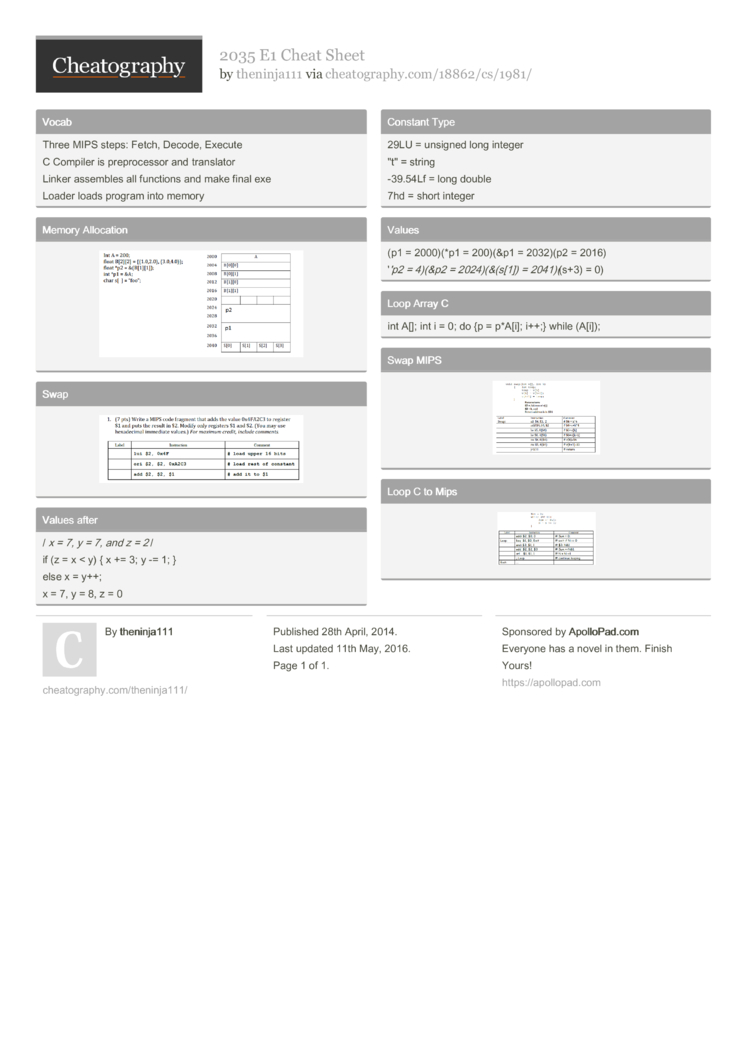# 2035 E1 Cheat Sheet by theninja111

Test

### Vocab

 Three MIPS steps: Fetch, Decode, Execute C Compiler is prepro­cessor and translator Linker assembles all functions and make final exe Loader loads program into memory

### Memory Allocation### Swap### Values after

 / x = 7, y = 7, and z = 2 / if (z = x < y) { x += 3; y -= 1; } else x = y++; x = 7, y = 8, z = 0

### Constant Type

 29LU = unsigned long integer "­t" = string -39.54Lf = long double 7hd = short integer

### Values

 (p1 = 2000)(*p1 = 200)(&p1 = 2032)(p2 = 2016) ''p2 = 4)(&p2 = 2024)(­&(­s) = 2041)(­(s+3) = 0)

### Loop Array C

 int A[]; int i = 0; do {p = p*A[i]; i++;} while (A[i]);

### Swap MIPS### Loop C to Mips1 Page
//media.cheatography.com/storage/thumb/theninja111_2035-e1.750.jpg

PDF (recommended)